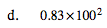### Home > MC2 > Chapter 10 > Lesson 10.1.6 > Problem10-76

10-76.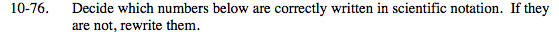Refer to the rules from the Math Notes box from Lesson 10.1.5:
-The first factor is less than 10 and greater than or equal to 1.
-The second factor has a base of 10 and an integer exponent.
-The factors are separated by a multiplication sign.

This is not written in scientific notation.
Which one of the rules is not followed in this notation?

The first factor is more than 10. We can move the decimal one digit to the left so that the number is between 1 and 10. How will that change the second factor?

By moving the decimal one digit to the left to make 9.25, we must add 1 to the exponent of 10. If we move the decimal tothe right, we must subtract 1 from the exponent of 10.

9.25 · 10−1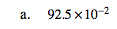Are any of the rules broken by this notation?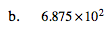Are all of the rules followed by this notation?

Yes. The second factor has a base of 10 and an integer exponent of 1.

101 = 10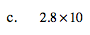The first and second rules for scientific notation are both broken. How can you rewrite each factor to satisfy both rules?

1002 is (100)(100). This value is 10,000. How many times do you have to multiply 10 by itself to get 10,000?

(10)(10)(10)(10) = 10,000, so 4 times.
Now we have 0.83 · 104.

We need to change 0.83 to a number between 1 and 10. Move the decimal point one digit to the right. What does this do to the exponent of 10?

We have to subtract 1 from the exponent of 10. So now we have:

8.3 · 103